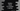# Python program to check if a number is prime or not## Python program to check if a number is prime or not:

In this post, we will learn how to check if a number is prime or not. A number is called a prime number if its factors are 1 and the number itself.

A number is a factor of another number if it can divide that number perfectly. Or, we can say that if the remainder is zero, then it is a prime number.

All prime numbers are greater than 1, i.e. 1 is not a prime number. So, the smallest prime number is 2.

We can check if a number is prime or not in different ways in Python. Let’s try them one by one.

## Method 1: Using a for loop:

We can use a for loop and that loop can find for any other number which can divide that given number perfectly. This loop will run from 2 to number - 1.

Below is the complete program:

``````num = int(input('Enter a number: '))

is_prime = True
for i in range(2, num):
if(num % i == 0):
is_prime = False
break

if is_prime == True:
print(f'{num} is a prime number')
else:
print(f'{num} is not a prime number')``````

Here,

• We are reading the number entered by the user and storing it in num.
• is_prime is initialized as True. This flag defines if the number is prime or not.
• The for loop runs from 2 to num - 1. For each value of i, we are dividing num by i and checking if the remainder is 0 or not. If it is zero, we are assigning is_prime False and also it exits from the loop.
• The last if else block prints if the number is prime or not.

If you run this program, it will print the below output:

``````Enter a number: 47
47 is a prime number

Enter a number: 48
48 is not a prime number``````

## Method 2: Using a for loop and iterating to num/2:

We don’t have to check up to the number in a loop. We can check up to number/2. Because, there can’t be any number greater than number/2 that can be a factor of number.

``````num = int(input('Enter a number: '))

is_prime = True
for i in range(2, int(num/2) + 1):
if(num % i == 0):
is_prime = False
break

if is_prime == True:
print(f'{num} is a prime number')
else:
print(f'{num} is not a prime number')``````

It will give similar output as the above example.

## Method 3: More optimization by iterating to the square root of the number:

We can optimize this further. We can check from 2 to square root of the number. Because, all factors of the number greater than √number should be a multiple of a number smaller than or equal to √number. So, if we iterate from 2 to √number, we can find if a number is prime or not.

``````from math import sqrt

num = int(input('Enter a number: '))

is_prime = True
for i in range(2, int(sqrt(num)) + 1):
if(num % i == 0):
is_prime = False
break

if is_prime == True:
print(f'{num} is a prime number')
else:
print(f'{num} is not a prime number')``````

It will print similar output.

``````Enter a number: 49
49 is not a prime number

Enter a number: 58
58 is not a prime number

Enter a number: 47
47 is a prime number``````